# 关注数学发展,弘扬科学精神,专注数学科普

## 中值定理之我见1 罗尔中值定理

1.1 直觉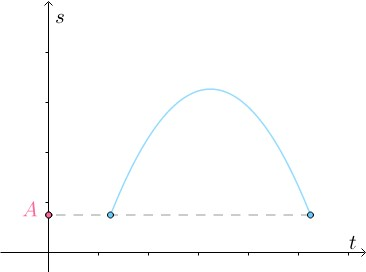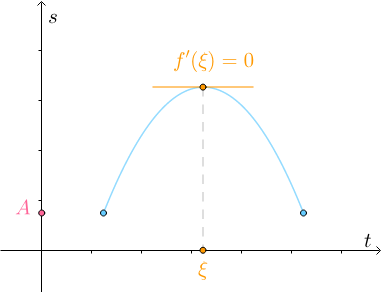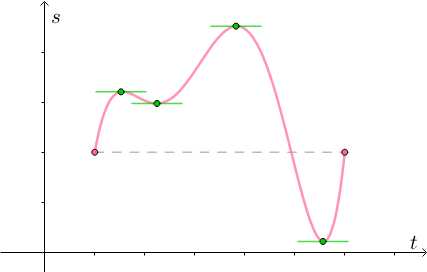1.2 罗尔中值定理

f(x) 在闭区间 [a,b] 上连续

• f(x) 在开区间 (a,b) 上可导

• f(a)= f(b)

f(x) 在闭区间 [a,b] 连续是必须的，否则有可能没有 f’(ξ)=0 ：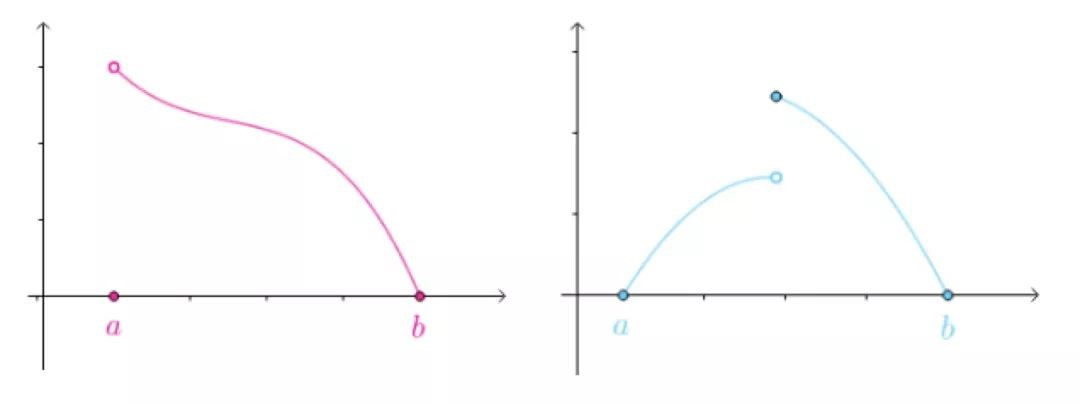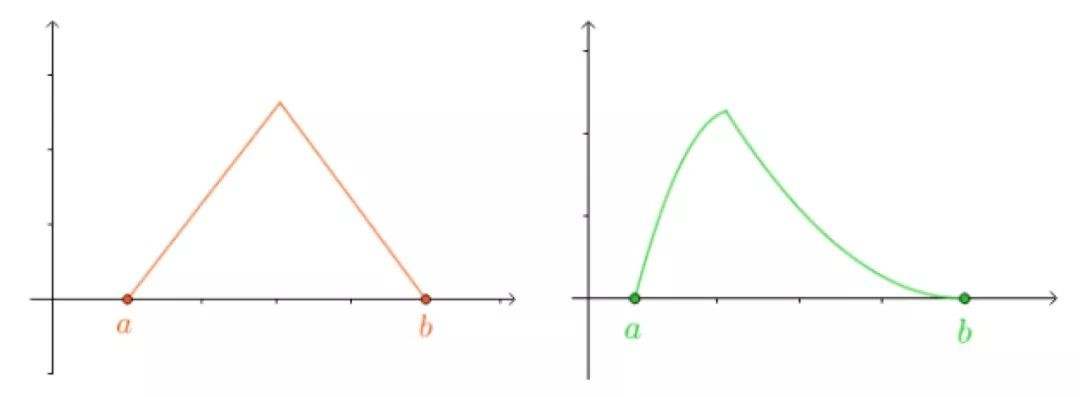### 1.3 拓展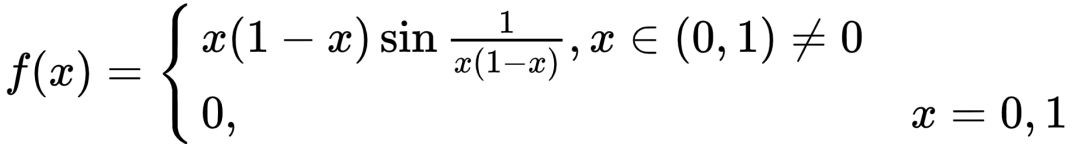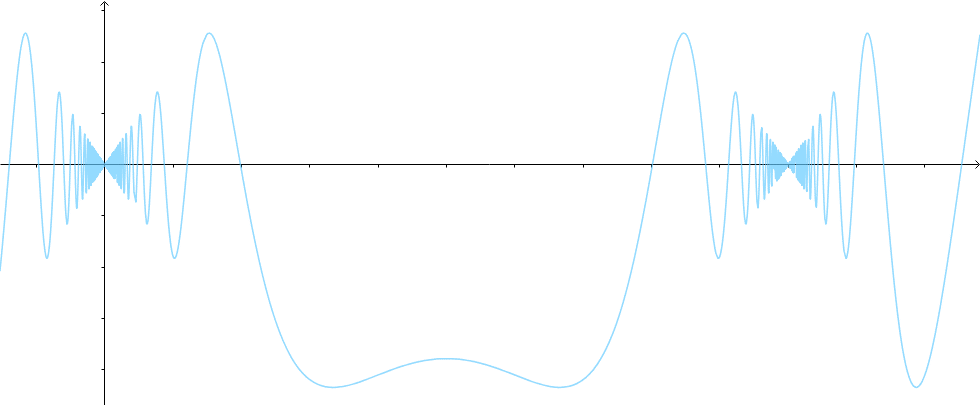2 拉格朗日中值定理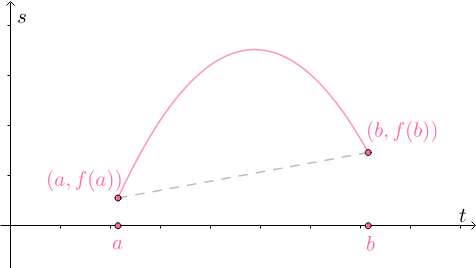• 匀速前进：那么整个路程的瞬时速度必然全为 70km/h

• 变速前进：整个路程的瞬时速度必然有大于、等于、小于 70km/h 的情况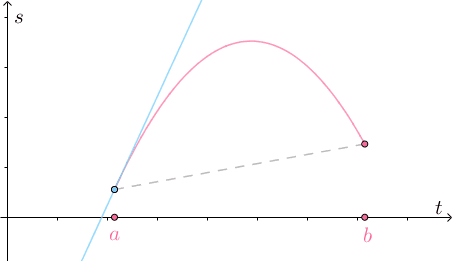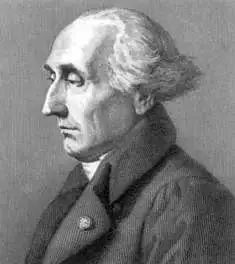2.1 拉格朗日中值定理

• f(x) 在闭区间 [a,b] 上连续

• f(x) 在开区间 (a,b) 上可导

f’(ξ)=[f(b)-f(a)]/(b-a)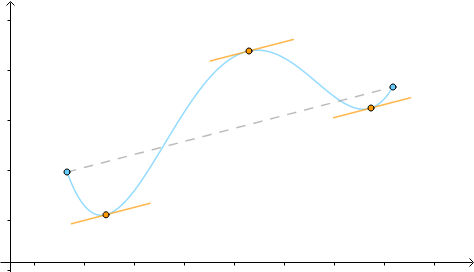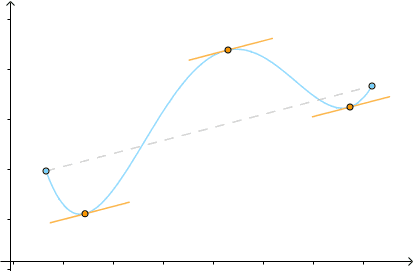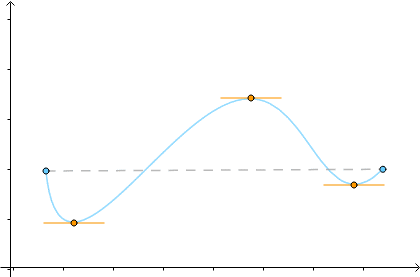3 柯西中值定理设函数

f(x),g(x) 满足以下条件：

• f(x),g(x) 在闭区间 [a,b] 上连续

• f(x),g(x) 在开区间 (a,b) 上可导

• ∀xϵ (a,b) 有：g’(x)≠0

[f(b)-f(a)]/[g(b)-g(a)]= f’(ξ)/ g’(ξ)

x=f(t)

y=g(t)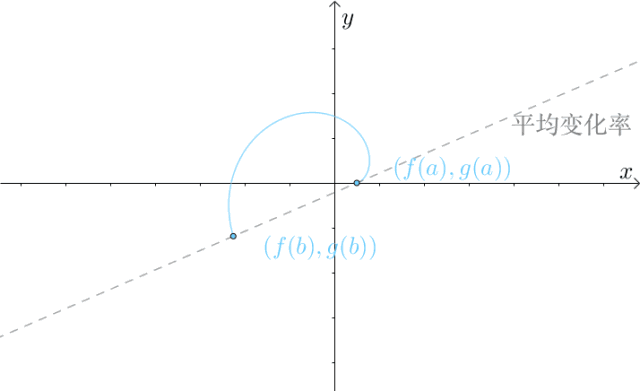x=x

y=f(x)

4 总结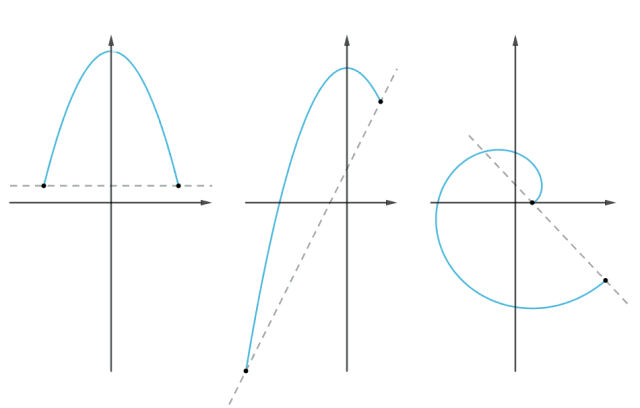（声明：本文仅代表作者观点，不代表本站观点，仅做陈列之用）

[责编：雨滴]